JOINProblem Statement

Problem: SuperResolution

### Problem Statement

Many modern cell phones have digital cameras built in to them. While this is quite convenient, the quality of the pictures leaves something to be desired. Low megapixel count and small apertures lead to pictures plagued by camera shake and low resolution. However, by taking many photos of the same scene, one can hope to post-process these images into a high-quality final image.

In this problem, you will be given many images of a single scene. Each of the image will be slightly blurred from movement of the camera, and will be rather low resolution. Your task is to reconstruct a high resolution image from these images.

To create test cases, we will start with a single high resolution image. We will consider the high resolution image to be ground truth, and thus imagine that our camera phone is taking a picture of a grid, each of whose 1x1 unit squares is a constant color (one pixel from the original image). Each of the camera phone pixels will be DxD units (where D is not necessarily an integer). Pixel (0,0) of the camera phone will start with its lower left corner at a random offset (offx,offy) where offx and offy are in [0,D). A point (shakex,shakey) will then be chosen, and the lower left corner of pixel (0,0) of the camera will move continuously (in a straight line) to (offx+shakex,offy+shakey). shakex and shakey will each be chosen from a normal distribution with mean 0 and standard deviation SD. The resulting color of each camera pixel will simply be the average of the colors under it during the movement (some cells will be partially covered by the pixel). The movement will be continuous at a constant rate. Thus, a move from (0,0) to (1,0) will be at (0.5,0) after half a time unit.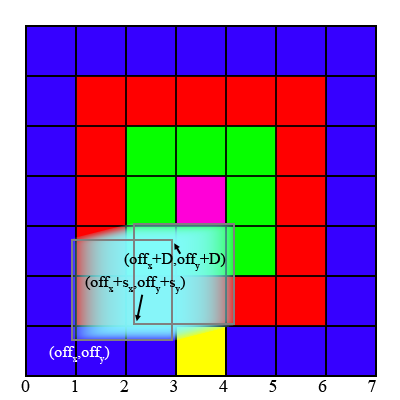This is illustrated in the image above. The original image is 7x7 pixels. Each camera pixel covers 2x2 of the pixels in the original image, so D=2.0. Note that although none of the final positions cover any of the yellow pixel, the large pixel is moving continuously, and thus covers part of the yellow pixel at some point in between the two positions. The scaled down image will be W'' = floor(W'/D)-1 pixels wide, where W' is the size of the high resolution image. The height will be similarly computed. To avoid effects from the edge of the image ceil(5*SD/D) pixels will be removed from each edge of the low resolution image before it is given to you, leaving it W = W''-2*ceil(5*SD/D) pixels wide, and similarly high. To ensure this is enough, if shakex or shakey has absolute value greater than 5*SD it will be discarded, and a new value chosen.

For each test case, the number of images, N, will be chosen in [10,100], the value of SD will be chosen (as a real) in [1,5] and the value of D will be chosen as a real in [4,20]. You will be given the images as a int[]. Each of the images will be W pixels wide, and H pixels high. Pixel (x,y) in image z (all indexed from 0) will be given by element W*H*z + W*y + x of images. Each pixel value is encoded as an integer with eight bits per color component in RGB order, from most significant to least significant. You can decode each pixel with int red = (pixel >> 16), green = (pixel >> 8) & 0xff, blue = pixel & 0xff.

You should return a int[] similarly formatted: each pixel should be encoded in the same way, in row major order. Your return should be retW = floor(W*D-50) pixels wide, and retH = floor(H*D-50) pixels high. To evaluate your image, we will consider all (x,y) offsets that place your return entirely within the region covered by the original image. Of all these offsets, we will pick the one that achieves the lowest squared error on a sampling of (roughly) 22,500 pixels as follows:
```    sampleSE(int offx, int offy){
DW = max(1,floor(W*D-50)/150)
DH = max(1,floor(H*D-50)/150)
for(int i = DW/2; i<retW; i+=DW){
for(int j = DH/2; j<retH; j+=DH){
//add SE between pixel (i,j) of your return and pixel (i+offx,j+offy) of the original
}
}
}
```
When we find the best squared error on this sample, we will then compute the minimum squared error over the entire image with 9 offsets: the one computed from the sample, and the 8 surrounding it (or less if some of them place your image outside the original image). (The squared error between two images is the sum over all pixels and color channels of the squared difference between the correct color value and the value you give. Your image will be smaller than the original image, and only the overlapping part will be considered). We will then compare your squared error to a baseline value. The baseline value will be calculated by considering retW x retH pixels in the center of the original image. For each pixel (x,y), the average color value of a box 2*ceil(D)+1 pixels will be computed, with (x,y) at the center of that box. We will compute the squared error between the original image, and these averages. Your score on a test will be your improvement over this baseline: (base-you)/base.

For each test case, you'll receive YOU/BEST points, where BEST is the best improvement out of all competitors, and YOU is your score on that test case.

A number of tools and files are available here.

### Definition

 Class: SuperResolution Method: upscale Parameters: int, int, int, int, double, int[] Returns: int[] Method signature: int[] upscale(int W, int H, int retW, int retH, double D, int[] images) (be sure your method is public)

### Notes

-The test data is generated from real images. Each image was shot using Nikon's raw file format (12 bits per channel) on a D50. The images were then converted to 8 bits/channel and the sRGB colorspace. The originals are all 3008 pixels wide, and 2000 pixels high or 3008 pixels high and 2000 wide. With the exception of some of the examples, all of the test images will be from this dataset. The examples are all processing in the same way, but some of them are cropped.
-The time limit is 90 seconds per test case.
-The memory limit is 2048M.
-The thumbnails here are downscaled images from provisional and final tests. They were randomly selected from the full set of test cases. Sixty-one of the images shown there were randomly selected as provisional tests.
-If your percent improvement is negative, it will be increased to 0 when computing the final score.
-The full size examples are all available for download at http://www.topcoder.com/contest/problem/SuperResolution/examples.tar

### Examples

0)

```D = 7.496623935331684
SD = 4.909701519772209
N = 60```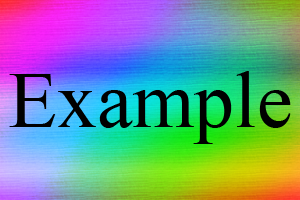The only simulated test case.
1)

```D = 19.30374801564643
SD = 4.002685612912828
N = 87```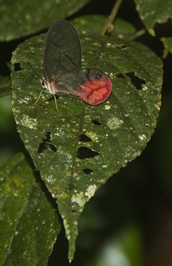Shown at 20%
2)

```D = 13.448703825640285
SD = 1.877371120265936
N = 77```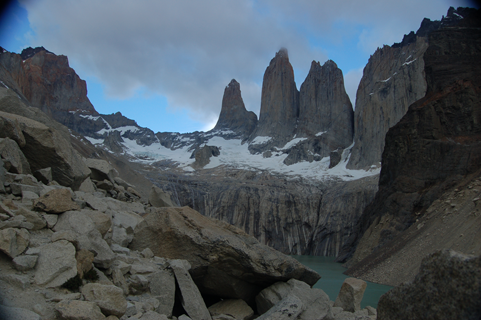Shown at 40%
3)

```D = 14.376319789341073
SD = 4.136449285874591
N = 66```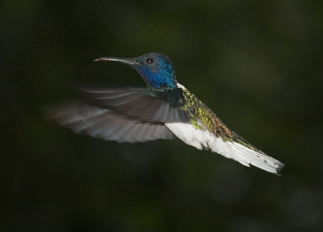Shown at 20%
4)

```D = 8.893131228228626
SD = 2.258001991663385
N = 96```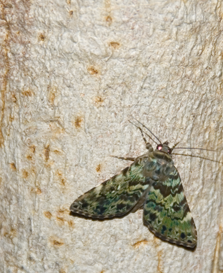Shown at 20%
5)

```D = 6.12897202244668
SD = 1.9863858962483674
N = 15```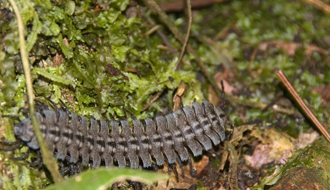Shown at 20%
6)

```D = 17.80275800197903
SD = 3.41687203289043
N = 24```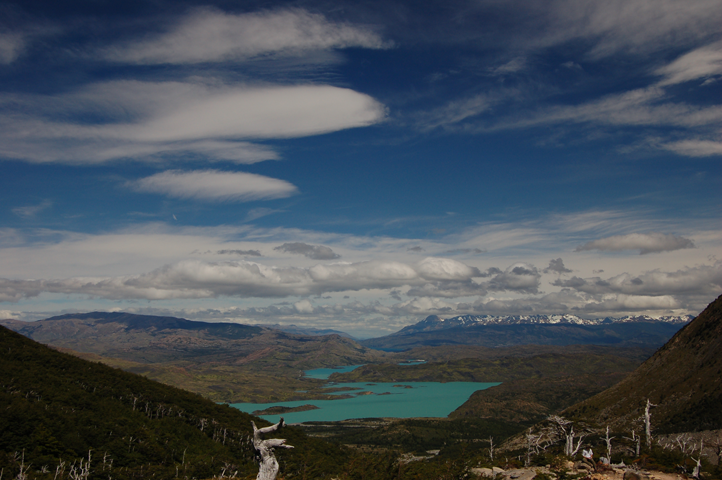Shown at 40%
7)

```D = 18.702298622724143
SD = 2.927506634551755
N = 14```Shown at 20%
8)

```D = 4.417301308597786
SD = 1.06886361236849
N = 15```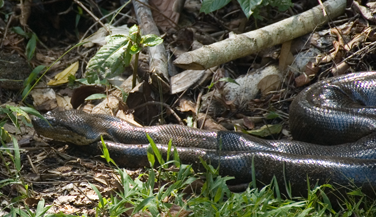Shown at 20%
9)

```D = 11.813562582372441
SD = 3.488679421696652
N = 99```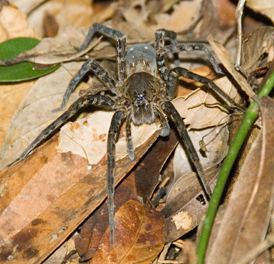Shown at 20%
10)

```D = 6.625634821586864
SD = 2.4309360880483544
N = 41```Shown at 20%
11)

```D = 10.455900973173662
SD = 1.9767848824149716
N = 52```Shown at 20%
12)

```D = 7.676215010962938
SD = 4.302102080146356
N = 70```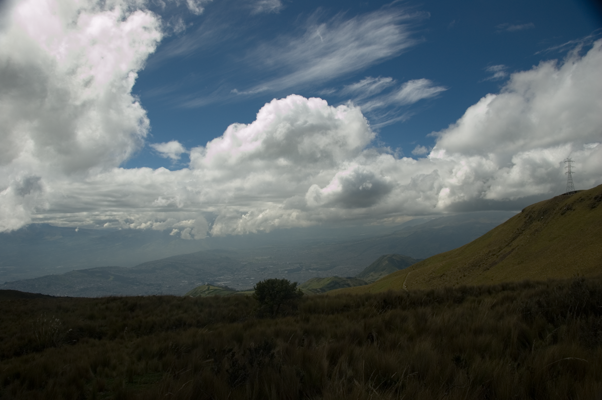Shown at 20%
13)

```D = 19.469413829204385
SD = 2.0160879278476664
N = 61```Shown at 20%
14)

```D = 11.315143769332504
SD = 2.9833704297712953
N = 86```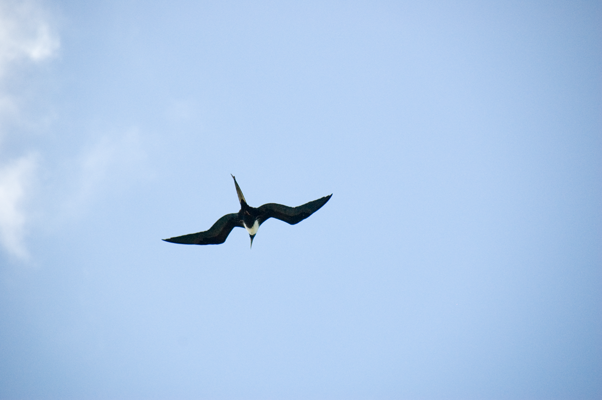Shown at 20%
15)

```D = 6.55351666175625
SD = 3.3045535534901815
N = 34```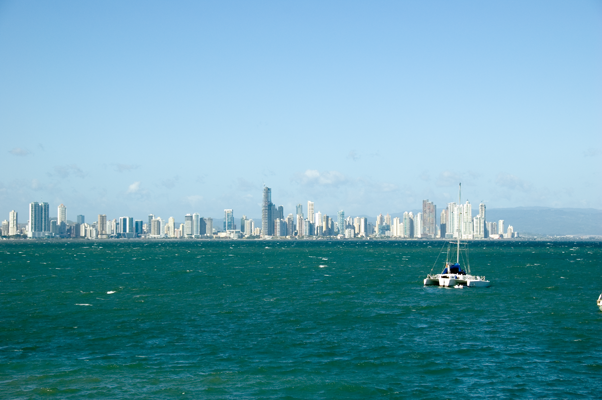Shown at 20%
16)

```D = 7.136091394557436
SD = 3.007810896371809
N = 56```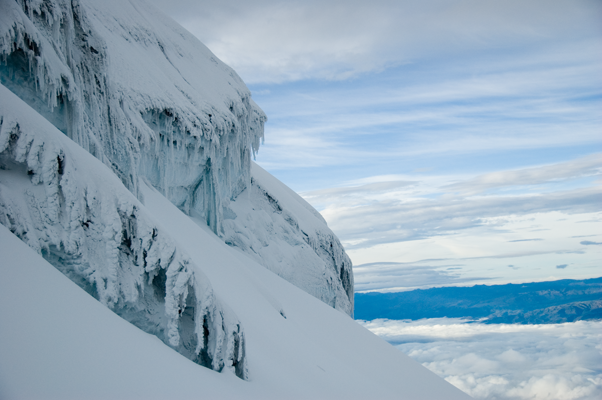Shown at 20%
17)

```D = 5.679655046950442
SD = 2.0429885920195203
N = 21```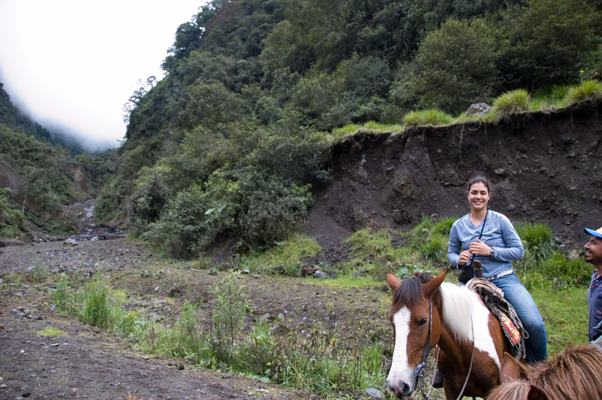Shown at 20%
18)

```D = 7.054607055262268
SD = 1.5827134294609984
N = 84```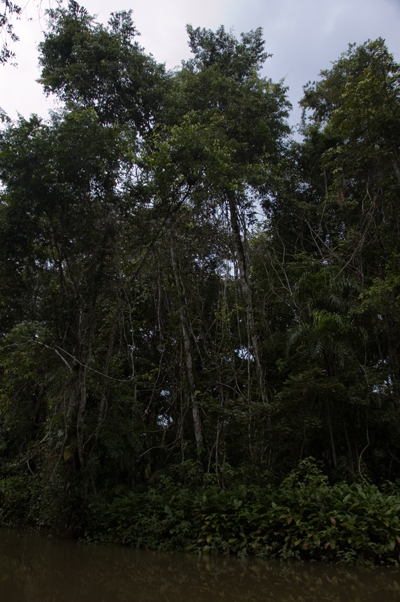Shown at 20%
19)

```D = 14.003517450053167
SD = 2.106091330950422
N = 79```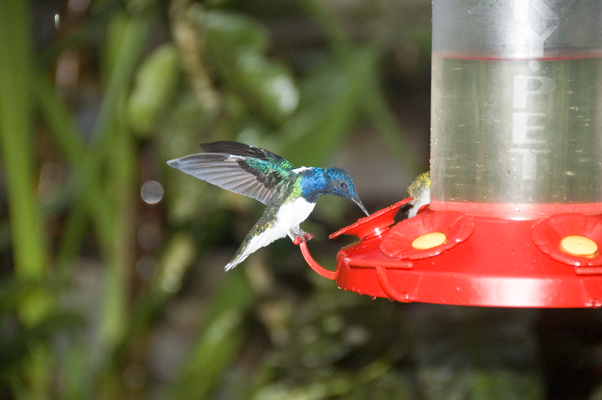Shown at 20%
20)

```D = 16.44493261367146
SD = 3.642567824158242
N = 23```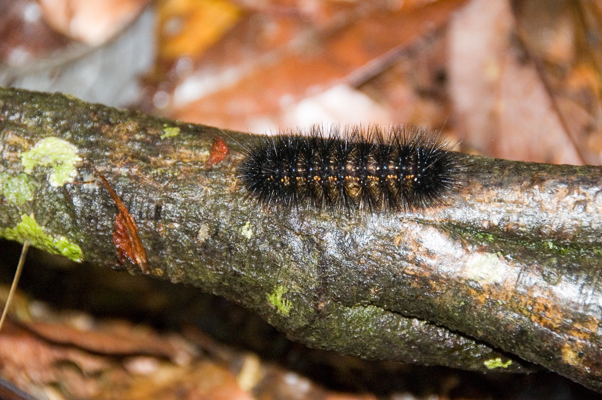Shown at 20%

This problem statement is the exclusive and proprietary property of TopCoder, Inc. Any unauthorized use or reproduction of this information without the prior written consent of TopCoder, Inc. is strictly prohibited. (c)2010, TopCoder, Inc. All rights reserved.